# Point sets with no empty pentagon

 Importance: Low ✭
 Author(s): Wood, David R.
 Subject: Geometry
 Keywords: combinatorial geometry visibility graph
 Posted by: David Wood on: December 14th, 2010
Problem   Classify the point sets with no empty pentagon.

Let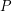be a finite set of points in the plane (not necessarily in general position). Two pointsare visible if the line segment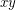contains no other point in. The visibility graph ofhas vertex set, where two vertices are adjacent if and only if they are visible. An empty pentagon inconsists of 5 points inthat are the vertices of a strictly convex pentagon whose interior contains none of the points in.

Consider the following three closely related classes of point sets: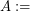point sets with no empty pentagon (called a 5-hole),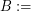point sets with no 5 pairwise visible points,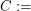point sets whose visibility graph is 4-colourable.

By definition,, and it is easy to show that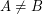and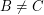.

A key example of a point set inis the planar grid (intersected with a convex set so that it is finite): colour each grid point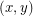by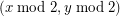. Ifand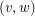receive the same colour thenand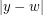are both even, and thus the midpoint ofandis a blocker. Hence the visibility graph of the grid is 4-colourable. [This result and proof is folklore.] Many other examples of point sets in these classes can be found in the references.

Consider the following open problems:

\item Classify the point sets in,, or(i.e. list all examples; this is easy for point sets with no empty quadrilateral, or no 4 pairwise visible points).
\item Does the visibility graph of every point set inhave bounded chromatic number?
\item Does the visibility graph of every point set inhave bounded clique number?
\item Does the visibility graph of every point set inhave bounded chromatic number?

Kára-Pór-Wood gave an example of a point set inwith chromatic number.

## Bibliography

Z. Abel, B. Ballinger, P. Bose, S. Collette, V. Dujmovic, F. Hurtado, S. D. Kominers, S. Langerman, A. Pór, D. R. Wood. Every large point set contains many collinear points or an empty pentagon, Graphs and Combinatorics 27(1):47-60, 2011.

Eppstein, David. Happy endings for flip graphs. J. Computational Geometry 1(1):3-28, 2010. MathSciNet

Kára, Jan; Pór, Attila; Wood, David R. On the chromatic number of the visibility graph of a set of points in the plane. Discrete Comput. Geom. 34(3):497-506, 2005. MathSciNet

Pfender, Florian. Visibility graphs of point sets in the plane. Discrete Comput. Geom. 39 (2008), no. 1-3, 455–459. MathSciNet

Rabinowitz, Stanley. Consequences of the pentagon property. Geombinatorics 14:208-220, 2005.

* indicates original appearance(s) of problem.

### Problem 3 solved

Cibulka, Kyncl and Valtr have solved problem 3. They constructed point sets with no empty pentagon, and whose visibility graph has arbitrarily large clique number.

Josef Cibulka, Jan Kyncl and Pavel Valtr: On planar point sets with the pentagon property, Proc. SoCG 2013, pp. 81-90. http://dl.acm.org/citation.cfm?id=2462406

### Problem 2 solved

Since the chromatic number is always equal or greater than the clique number, the chromatic number of the visibility graph of sets in A is also unbounded.

Cibulka, Kyncl and Valtr have also indirectly solved problem 2.